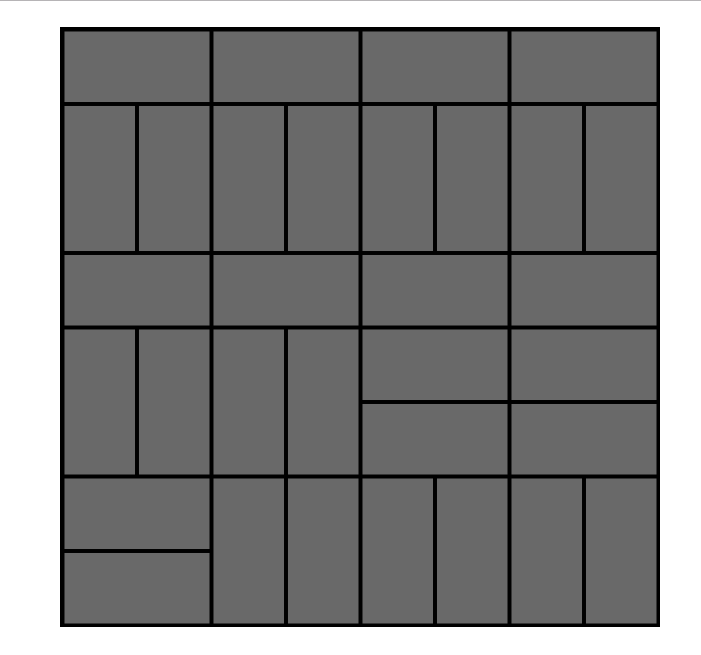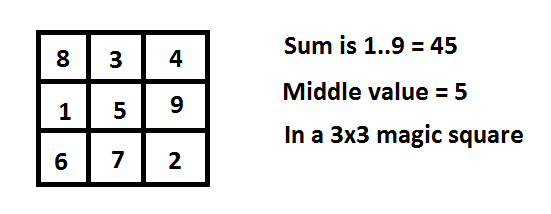# Monday September 18th

## Thought I’d include pics from my most recent daysail.

• Sea lions!• Coming back in. I was tasked with tying off at the bow.• Our post meal of prosecco, caprese and tomatoes. We also had smoked oysters!## Things to do

• Coding test (done)

• Haskell course start (done with Week 1)

• Continue with C (done with part 1..start on crypto assignment (2 and 3))

• Nanodegree videos finish (rest of week work on)

## Katas

• Format code so matches result
``````def list_animals(animals):
list = ''
for i in (animals):
list += str(animals.index(i)+1) + '. ' + str(i) + '\n'

return list
``````
• If there is a gold in the array, return True, else False
``````def check_the_bucket(bucket):
total = 0
for i in bucket:
if i == 'gold':
total = total + 1
if total == 0:
return False
else:
return True
``````
• Find unique item in a list
``````def find_uniq(arr):
# find all unique items
a = list(set(arr))
# find count of unique item in orig list
for i in a:
# if count of item is 1, that is unique item
if arr.count(i) == 1:
return i
``````
• see if input matches type
``````function typeValidation(variable, type) {
if (typeof(variable) == type){
return true
}
else{
return false
}
}
``````
• given two arrays eg [1,2,3] and [1,2]
• 123 + 12 = 243 = [2,4,3]
``````function addArrays(array1, array2) {
var arrayToNumber1 = parseInt(array1.join(''));
var arrayToNumber = parseInt(array2.join(''));
var arr3 = arrayToNumber1 + arrayToNumber;
var arr4 = (arr3.toString())
arr = arr4.split("")
var final = []
for (var i = 0; i < arr.length; i++){
final.push(parseInt(arr[(i)]))
}
return final
}
``````

## Things of interest

• Interesting Knuth video
• includes McGregor graphs, Colored Queens problem, Sat Solvers
• Proof solution to whether 2x1 domino can cover 8x8 board• Eulerian path ..used in determining Tensegrity. Visit every path exactly once

• Magic Square

• I found this solution first, before understanding the mathematics behind it. My biggest question was how to figure out the total sum of each row/column/diagonal. Very interesting, and from Coursera’s “Intro to Proofs” course.• Another puzzle
``````"""
there is a 6 digit
number starting 100
and is divisible by 9127
"""

total = 0
for i in range(100000,100999):
if i % 9127 == 0:
total = i
print total